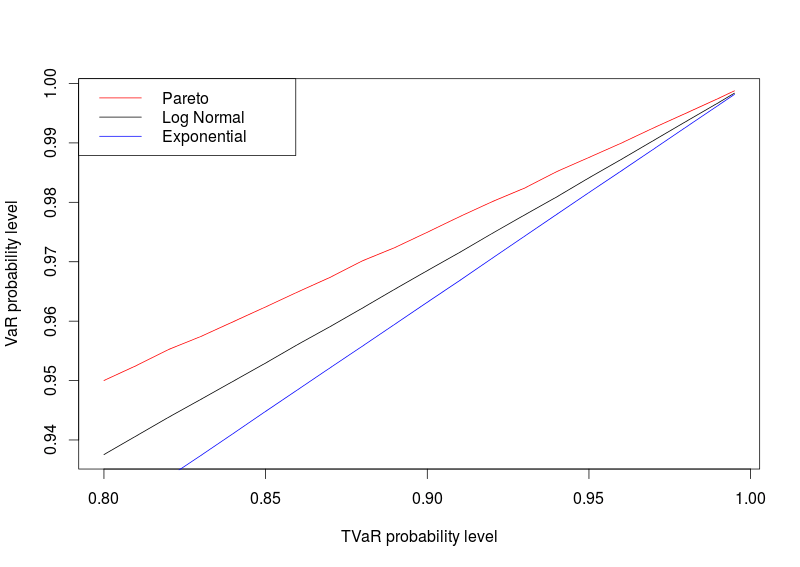# “A 99% TVaR is generally a 99.6% VaR”

August 29, 2015
By

Want to share your content on R-bloggers? click here if you have a blog, or here if you don't.

Almost 6 years ago, I posted a brief comment on a sentence I found surprising, by that time, discovered in a report claiming that

the expected shortfall […] at the 99 % level corresponds quite closely to the […] value-at-risk at a 99.6% level

which was inspired by a remark in Swiss Experience report,

expected shortfall […] on a 99% confidence level […} corresponds to approximately 99.6% to 99.8% Value at Risk

Recall that

while

For any (absolutely) continuous cumulative distribution function, strictly increasing, since both (the VaR and the TVaR) are continuous, and strictly increasing, it is possible to relate any TVaR to some VaR, with a different level.  I.e.

Which is not the same as

Consider for instance the lognormal distribution. Since there is no simple expression for the expected shortfall, use monte carlo simulation to approximate it. And then, use the cumulative distribution function to get the assocated level for the value at risk,

```> n=1e7
> TVaR_VaR_LN=function(p){
+     X=rlnorm(n)
+     E=mean(X[X>qlnorm(p)])
+     return(plnorm(E))
+ }```

E.g.

```> TVaR_VaR_LN(.99)
 0.9967621```

In order to plot it, define

```> prob=c(seq(.8,.99,by=.01),.995)
> P_ln=unlist(lapply(prob,TVaR_VaR_LN))```

Now, if we consider a distribution with lighter tails, like the exponential distribution

```> TVaR_VaR_exp=function(p){
+     X=rexp(n)
+     E=mean(X[X>qexp(p)])
+     return(pexp(E))
+ }
> P_exp=unlist(lapply(prob,TVaR_VaR_exp))```

or a distribution with heavier tails, like the Pareto one,

```> qpareto=function(u,a=2){(1-u)^(-1/a)}
> rpareto=function(n,a=2){qpareto(runif(n),a)}
> ppareto=function(x,a=2){1-1/x^a}
> TVaR_VaR_par=function(p){
+     X=rpareto(n)
+     E=mean(X[X>qpareto(p)])
+     return(ppareto(E))
+ }
> P_pareto=unlist(lapply(prob,TVaR_VaR_par))```

we have different probability levels.

```> plot(prob,P_ln,type="l",xlab="TVaR probability level",ylab="VaR probability level")
> lines(prob,P_pareto,type="l",col="red")
> lines(prob,P_exp,type="l",col="blue")
> legend("topleft",
+        c("Pareto","Log Normal","Exponential"),
+        col=c("red","black","blue"),lty=1)```Hence, the heavier the tail, the higher the probability level. So, always qppfoximating a 99% TVaR with 99.6% VaR might work in some cases, e.g.

```> TVaR_VaR_exp(.99)
 0.9963071```

but I is usually an optimistic approximation.

R-bloggers.com offers daily e-mail updates about R news and tutorials about learning R and many other topics. Click here if you're looking to post or find an R/data-science job.
Want to share your content on R-bloggers? click here if you have a blog, or here if you don't.